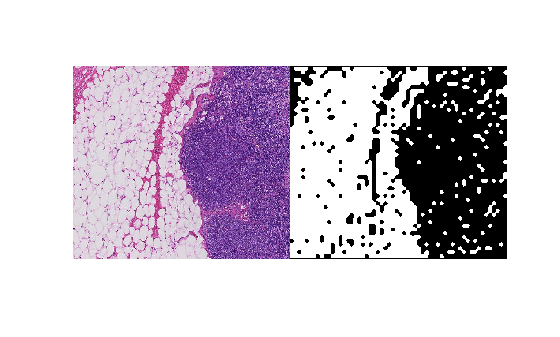sub2world

Convert pixel subscripts to block subscripts

Description

example

world = sub2world(bim,pixelsub) converts the pixel subscripts, pixelsub, to the block subscript of the block containing the corresponding pixel.

world = sub2world(bim,pixelsub,'Level',L) additionally specifies the resolution level to use in a multiresolution image. Level defaults to 1.

Examples

collapse all

Convert pixel subscripts from one level to another via the world coordinates to refer to the same spatial region.

Create a blocked image from a sample image included with the toolbox.

bim = blockedImage('tumor_091R.tif');

Define a region of interest in the finest resolution level in pixel subscripts.

level1PixelSubStart = [1700, 1550 1];
level1PixelSubEnd = [2100, 2000 3];

Get the image data from the region of interest on the resolution level 1 image.

imr = getRegion(bim, level1PixelSubStart, level1PixelSubEnd, "Level", 1);
size(imr)
ans = 1×3

401   451     3

Convert the pixel subscripts that define the region of interest into world coordinates. By default, sub2world converts the coordinates at level 1, the finest resolution.

worldRegion = sub2world(bim,[level1PixelSubStart; level1PixelSubEnd]);

Compute a binary mask at the coarsest level.

bbw = apply(bim, @(bs)imbinarize(im2gray(bs.Data)), "Level", 3);

Convert the world coordinates of the region of interest to pixel subscripts of the mask. Note that the mask has only two dimensions.

worldRegion = worldRegion(:,1:2);

size(bwr)
ans = 1×2

51    58

View the original image and the mask.

montage({imr,bwr})Input Arguments

collapse all

Blocked image, specified as a blockedImage object.

Pixel subscripts, specified as a K-by-N integer-valued vector, where N is the number of dimensions and K is the number of coordinates.

Output Arguments

collapse all

World subscripts, returned as a K-by-X numeric array. The world coordinates are in the same order as the pixel subscripts. For an N-dimensional blockedImage, and K subscripts (each row is a separate subscript), pixelsub is a K-by-N integer-valued matrix and world is a K-by-N numeric matrix.